# 交流电路中的被动元件单击或点击下面的示例电路以调用TINACloud并选择交互式DC模式以在线分析它们。

VM = R *IM or V = R *I

VM = R * IM or V = R * I.1. 对于 高峰 (VM，我M ），或 有效 当前和的（V，I）值 电压：

VM = XL*IM or V = X.L*I

2. 使用复杂的相量：

VM = j * XL IM or V = j * XL * I

ZL= V/I = VM / IM = j w L

YL= I / V = IM /VM = 1 /（j w L)

VM = XL IM or V = X.L * 一世

 证明 纯线性电压的时间函数 感应器 通过考虑与电感器的电压和电流相关的时间函数，可以找到（内部电阻为零且无杂散电容的电感器）：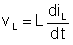. 使用上一章介绍的复杂时间函数概念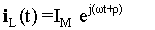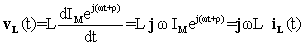使用复杂的相量： VL = j w L* IL 或具有实时功能 vL （t）= w L iL （T + 90°) 所以电压是90° 在当前之前。

ILM= VLM/(jwL）= 1 /（j6.28 100 * * 0.001）= - j1.59A

iL（t）= 1.59sin（wT-90°） 一个。#### 例如1

XL = 2 *p* f * L = 2 * 3.14 * 50 * 0.003 = 0.9425欧姆= 942.5欧姆

ZL= j w L = j 0.9425 = 0.9425 j 欧姆#### 例如2

XL = 2 *p* f * L = 2 * 3.14 * 200 * 3 = 3769.91欧姆° 背后 （在一个 逆时针方向）的电流。 在复平面中，电压相量在负方向（相对于参考方向，逆时针）上垂直于电流相量。 您可以使用虚数以复数来表示–j 作为乘数。

1。 为了 绝对峰值 or 有效 当前和的价值 电压：or V = X.C*I

2。 为了 复杂的高峰 or 有效 电流和电压值：

VM = –j * XC*IM or V = – Ĵ* XC*I

ZC = V / I = VM / IM = – j*XC = – j / wC

YC= I / V = IM / VM = j wC)

 证明： 常规产品的 纯线性电容上的电压的时间函数（无并联或串联电阻且无杂散电感的电容器） 可以用电容器电压的时间函数表示（vC），费用（qC）和当前（iC ):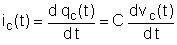如果C不依赖于时间，则使用复杂的时间函数： iC（t）= j w C vC（T） or vC（t）=（ - 1 /jwC）*iC（T） 或使用复数相量：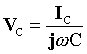或具有实时功能 vc （t）= ic （叔90°）/（w C) 所以电压是90° 背后 目前。

ICM= jwCVCM =j6.28 * 10 610-7 * 2）=j1.26，

iL（t）= 1.26sin（wT + 90°）一个

#### 例如3

XC = 1 /（2 *p*f*C) = 1/(2*3.14*50*25*10-6）= 127.32欧姆

Z-C= 1 /（j w C）= – j 127.32 = –127.32 j 欧姆XC = 1 /（2 *p*f*C) = 1/(2*3.14*200*103* * 25 10-6）= 0.0318 = 31.8 mohms。Z = V / I = VM/IM

ZR = R; ZL = j w L ZC = 1 /（j w C）= –j / wC

X欢迎来到 DesignSoft
如果需要任何帮助找到合适的产品或需要支持，可以进行聊天。